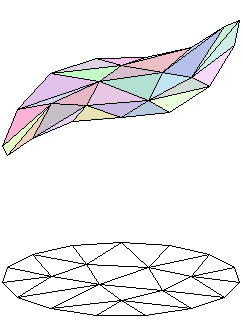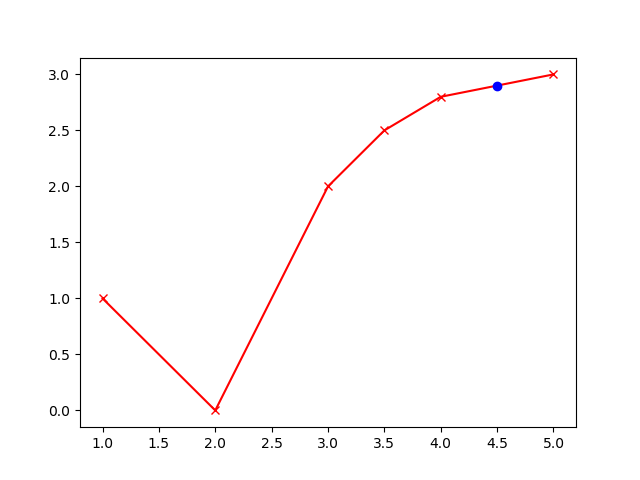## Piece-wise Linear ApproximationA piece-wise linear function is an approximation of a nonlinear relationship. For more nonlinear relationships, additional linear segments are added to refine the approximation.

As an example, the piecewise linear form is often used to approximate valve characterization (valve position (% open) to flow). This is a single input-single output function approximation.

In Situ Adaptive Tabulation (ISAT) is an example of a multi-dimensional piecewise linear approximation. The piecewise linear segments are built dynamically as new data becomes available. This way, only regions that are accessed in practice contribute to the function approximation.#### APMonitor PWL Object

File p.txt
1,   1
2,   0
3,   2
3.5, 2.5
4,   2.8
5,   3
End File

Objects
p = pwl
End Objects

Connections
x = p.x
y = p.y
End Connections

Parameters
! independent variable
x = 6.0
End Parameters

Variables
! dependent variable
y
End Variables

#### Equivalent Problem with Slack Variables

Parameters
! independent variable
x = 6.0

! data points
xp = 1
yp = 1

xp = 2
yp = 0

xp = 3
yp = 2

xp = 3.5
yp = 2.5

xp = 4
yp = 2.8

xp = 5
yp = 3
End Parameters

Variables
! piece-wise linear segments
x             <=xp
x[2:4] >xp[2:4], <=xp[3:5]
x   >xp

! dependent variable
y

! slack variables
slk_u[1:4]
slk_l[2:5]
End Variables

Intermediates
slope[1:5] = (yp[2:6]-yp[1:5]) / (xp[2:6]-xp[1:5])
y[1:5] = (x[1:5]-xp[1:5])*slope[1:5]
End Intermediates

Equations
minimize slk_u[1:4]
minimize slk_l[2:5]

x = x   + slk_u
x = x[2:4] + slk_u[2:4] - slk_l[2:4]
x = x                - slk_l

y = yp + y + y + y + y + y
End Equations

#### PWL in GEKKO Pythonimport matplotlib.pyplot as plt
from gekko import GEKKO
import numpy as np

m = GEKKO()
m.options.SOLVER = 1

xz = m.FV(value = 4.5)
yz = m.Var()

xp_val = np.array([1, 2, 3, 3.5,   4, 5])
yp_val = np.array([1, 0, 2, 2.5, 2.8, 3])
xp = [m.Param(value=xp_val[i]) for i in range(6)]
yp = [m.Param(value=yp_val[i]) for i in range(6)]

x = [m.Var(lb=xp[i],ub=xp[i+1]) for i in range(5)]
x.lower = -1e20
x[-1].upper = 1e20

# Variables
slk_u = [m.Var(value=1,lb=0) for i in range(4)]
slk_l = [m.Var(value=1,lb=0) for i in range(4)]

# Intermediates
slope = []
for i in range(5):
slope.append(m.Intermediate((yp[i+1]-yp[i]) / (xp[i+1]-xp[i])))

y = []
for i in range(5):
y.append(m.Intermediate((x[i]-xp[i])*slope[i]))

for i in range(4):
m.Obj(slk_u[i] + slk_l[i])

m.Equation(xz == x   + slk_u)
for i in range(3):
m.Equation(xz == x[i+1] + slk_u[i+1] - slk_l[i])
m.Equation(xz == x                - slk_l)

m.Equation(yz == yp + y + y + y + y + y)

m.solve()

plt.plot(xp,yp,'rx-',label='PWL function')
plt.plot(xz,yz,'bo',label='Data')
plt.show()

#### Piece-wise Function with Lookup Object

! Piece-wise function with the LOOKUP object

! create the csv file
File m.csv
input,  y,  y
1,     2,    4
3,     4,    6
5,    -5,   -7
-1,     1,  0.5
End File

! define lookup object m
Objects
m = lookup
End Objects

! connect m properties with model parameters
Connections
x = m.input
y = m.y
y = m.y
End Connections

! simple model
Model n
Parameters
x = 1
y
y
End Parameters

Variables
y
End Variables

Equations
y = y+y
End Equations
End Model